## Exercises

1. Consider the set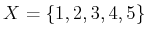. Let,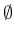,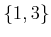,,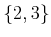,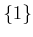,, and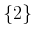be the collection of all subsets ofthat are designated as open sets.
1. Isa topological space?
2. Is it a topological space if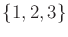is added to the collection of open sets? Explain.
3. What are the closed sets (assumingis included as an open set)?
4. Are any subsets ofneither open nor closed?

2. Continuous functions for the strange topology:
1. Give an example of a continuous function,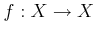, for the strange topology in Example 4.4.
2. Characterize the set of all possible continuous functions.

3. For the letters of the Russian alphabet, A, B, V, G, D, E, Ë, Zh, Z, I, uI, K, L, M, N, O, P, R, S, T, U, F, H, Ts, Ch, Sh, Shch, , Y, , E1, Yu, Ya, determine which pairs are homeomorphic. Imagine each as a 1D subset of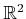and draw them accordingly before solving the problem.

4. Prove that homeomorphisms yield an equivalence relation on the collection of all topological spaces.

5. What is the dimension of the C-space for a cylindrical rod that can translate and rotate in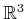? If the rod is rotated about its central axis, it is assumed that the rod's position and orientation are not changed in any detectable way. Express the C-space of the rod in terms of a Cartesian product of simpler spaces (such as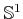,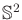,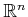,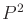, etc.). What is your reasoning?

6. Let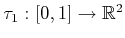be a loop path that traverses the unit circle in the plane, defined as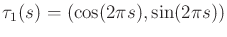. Let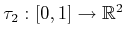be another loop path: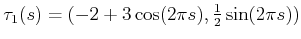. This path traverses an ellipse that is centered at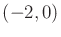. Show that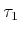and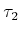are homotopic (by constructing a continuous function with an additional parameter that morphs''into).

7. Prove that homotopy yields an equivalence relation on the set of all paths from some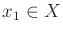to some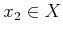, in which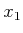and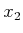may be chosen arbitrarily.

8. Determine the C-space for a spacecraft that can translate and rotate in a 2D Asteroids-style video game. The sides of the screen are identified. The top and bottom are also identified. There are no twists'' in the identifications.

9. Repeat the derivation of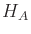from Section 4.3.3, but instead consider Type VE contacts.

10. Determine the C-space for a car that drives around on a huge sphere (such as the earth with no mountains or oceans). Assume the sphere is big enough so that its curvature may be neglected (e.g., the car rests flatly on the earth without wobbling). [Hint: It is not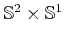.]

11. Suppose that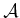and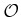are each defined as equilateral triangles, with coordinates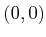,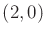, and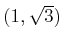. Determine the C-space obstacle. Specify the coordinates of all of its vertices and indicate the corresponding contact type for each edge.

12. Show that (4.20) is a valid rotation matrix for all unit quaternions.

13. Show that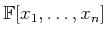, the set of polynomials over a field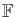with variables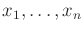, is a group with respect to addition.

14. Quaternions:
1. Define a unit quaternion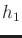that expresses a rotation of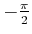around the axis given by the vector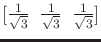.
2. Define a unit quaternion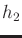that expresses a rotation of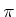around the axis given by the vector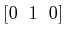.
3. Suppose the rotation represented byis performed, followed by the rotation represented by. This combination of rotations can be represented as a single rotation around an axis given by a vector. Find this axis and the angle of rotation about this axis.

15. What topological space is contributed to the C-space by a spherical joint that achieves any orientation except the identity?

16. Suppose five polyhedral bodies float freely in a 3D world. They are each capable of rotating and translating. If these are treated as one'' composite robot, what is the topology of the resulting C-space (assume that the bodies are not attached to each other)? What is its dimension?

17. Suppose a goal region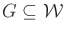is defined in the C-space by requiring that the entire robot is contained in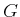. For example, a car may have to be parked entirely within a space in a parking lot.
1. Give a definition of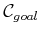that is similar to (4.34) but pertains to containment ofinside of.
2. For the case in whichandare convex and polygonal, develop an algorithm for efficiently computing.

18. Figure 4.29a shows the Möbius band defined by identification of sides of the unit square. Imagine that scissors are used to cut the band along the two dashed lines. Describe the resulting topological space. Is it a manifold? Explain.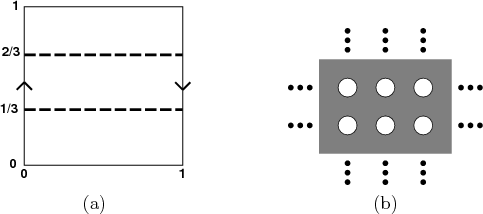19. Consider Figure 4.29b, which shows the set of points inthat are remaining after a closed disc of radius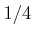with center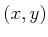is removed for every value ofsuch thatand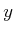are both integers.
1. Is the remaining set of points a manifold? Explain.
2. Now remove discs of radius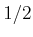instead of. Is a manifold obtained?
3. Finally, remove disks of radius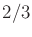. Is a manifold obtained?
20. Show that the solution curves shown in Figure 4.26 correctly illustrate the variety given in (4.73).
21. Find the number of faces of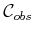for a cube and regular tetrahedron, assumingis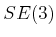. How many faces of each contact type are obtained?
22. Following the analysis matrix subgroups from Section 4.2, determine the dimension of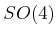, the group of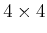rotation matrices. Can you characterize this topological space?

23. Suppose that a kinematic chain of spherical joints is given. Show how to use (4.20) as the rotation part in each homogeneous transformation matrix, as opposed to using the DH parameterization. Explain why using (4.20) would be preferable for motion planning applications.

24. Suppose that the constraint that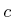is held to position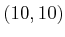is imposed on the mechanism shown in Figure 3.29. Using complex numbers to represent rotation, express this constraint using polynomial equations.

25. The Tangle toy is made of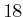pieces of macaroni-shaped joints that are attached together to form a loop. Each attachment between joints forms a revolute joint. Each link is a curved tube that extends aroundof a circle. What is the dimension of the variety that results from maintaining the loop? What is its configuration space (accounting for internal degrees of freedom), assuming the toy can be placed anywhere in?

Implementations

26. Computing C-space obstacles:
1. Implement the algorithm from Section 4.3.2 to construct a convex, polygonal C-space obstacle.
2. Now allow the robot to rotate in the plane. For any convex robot and obstacle, compute the orientations at which the C-space obstacle fundamentally changes due to different Type EV and Type VE contacts becoming active.
3. Animate the changing C-space obstacle by using the robot orientation as the time axis in the animation.
27. Consider straight-line'' paths that start at the origin (lower left corner) of the manifolds shown in Figure 4.5 and leave at a particular angle, which is input to the program. The lines must respect identifications; thus, as the line hits the edge of the square, it may continue onward. Study the conditions under which the lines fill the entire space versus forming a finite pattern (i.e., a segment, stripes, or a tiling).

Steven M LaValle 2020-08-14